Creating Quadratic Equations Given the Solution or the Graph MathBitsNotebook.com Terms of Use   Contact Person: Donna RobertsCan you determine the equation of a quadratic given its solutions?
If you are given the solutions of an equation, you can find an equation by working backward.
Let's see what happens when we use this strategy.
1. a. Determine an equation that has the solutions x = -4 and x = 3.
Work backward to find the equation: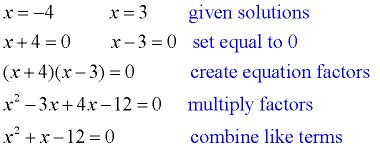We now have an equation answer:
x2 + x - 12 = 0
1. b. Find the solutions for the equation 3x2 + 3x - 36 = 0.

This equation can be solved by factoring.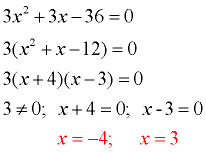Conclusion: Working backward will create an equation, but remember that there are other equations that will also have that same set of solutions. (Compare the solutions in 1a with 1b, and take a look at the graph at the right.) Any equation of the form k(x2 + x - 12) = 0, where k is a constant, has the same solutions as x2 + x - 12 = 0.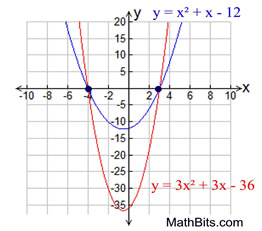Can we use this same strategy with complex solutions?
2. a. Determine an equation that has the solutions x = 2 + 3i and x = 2 - 3i.

Work backward to find the equation: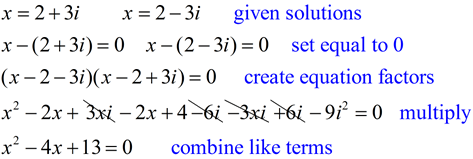An equation that has these roots is
x2 - 4x + 13 = 0

2. b. Does the equation 2x2 - 8x + 26 = 0 have these same roots?     YES!!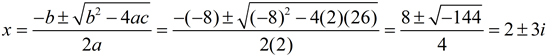Conclusion: As we saw in example 1, there are other equations that will also have that same set of solutions. (Compare the solutions in 2a with 2b.) Unlike example 1, however, it is not obvious from looking at the graphs of these equations that their roots are the same, since they do not cross the x-axis. Again, we see that any equation of the form k(x2 - 4x + 13) = 0, where k is a constant, has the same complex roots as x2 - 4x - 13 = 0.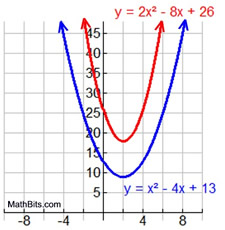If you have the graph of a parabola, can you determine the
equation of the quadratic function that created the graph?
Let's see what's possible.Graph with readable roots 3. Given the naked parabolic graph at the right, you are told that the x-intercepts and y-intercept are integer values. Thankfully, the graph shows a scale where each displayed unit on the x and y axes represents one unit. Since we know that the x-intercepts are integer values, we can read them off the graph to be (-3,0) and (4,0).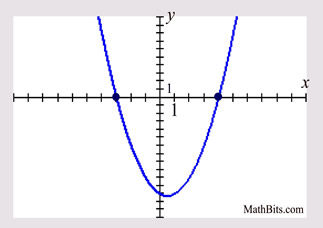If the x-intercepts are (-3,0) and (4,0), we know that the roots (zeros) of the equation will be x = -3 and x = 4. Working backward, we can create the factors (x + 3) and
(x - 4) and get the equation y = (x + 3)(x - 4).
BUT... do we know for sure that there is no numeric factor in this equation? Could it be, for example, y = 2(x + 3)(x - 4), since this equation also has zeros of x = -3 and x = 4 ?
We need to check another point. The only observable point is the y-intercept (0,-12).
Since (0,-12) is on this graph, it will satisfy the equation of the graph.
 Our First Equation: y = (x + 3)(x - 4). -12 = (0+ 3)(0 - 4). -12 = -12 TRUE Possible Second Equation: y = 2(x + 3)(x - 4) -12 = 2(0 + 3)(0 - 4) -12 ≠-24 FALSE Our first equation is the answer: y = (x + 3)(x - 4) or y = x2 - x - 12 .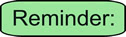The zeros obtained from the x-intercepts of a graph can determine the equation of a "family" of graphs. But, ONE MORE POINT is needed to guarantee a specific, individual function's equation.Graph with vertex and point 4. Given the parabolic graph at the right, where it is known that the turning point is (2,-3) and another random point on the graph is (5,6). Write the equation of the function which created the graph. It does not appear that the roots (zeros) of this parabola cross the x-axis at integer values, so the approach we used in the example above will not work here.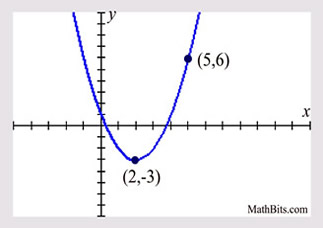We will use the vertex form: y = a(x - h)2 + k. We know the vertex is (2,-3) = (h,k), so h = 2 and k = -3. If we substitute, we get y = a(x - 2)2 + (-3).
Now, what about "a" ? We know that the point (5,6) is on the graph, which means that if we substitute x = 5 into the graph's equation, we will get a y-value of 6.
 y = a(x - 2)2 + (-3) 6 = a(5 - 2)2 + (-3) 6 = 9a - 3 9 = 9a 1 = a The value for a in this problem is "1". The equation is: y = (x - 2)2 + (-3).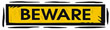You cannot assume that the a-value will always be 1.Graph with 3 distinct points 5. Given the parabolic graph at the right, where three points are given: (2,6), (1,-1) and (-3,-9). Write the equation of the function which created the graph. The exact locations of the roots and the vertex are not clear from examining the graph.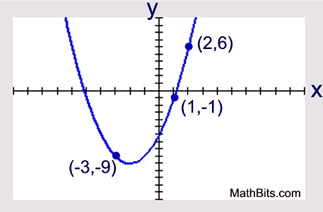We will use the quadratic form: y = ax2 + bx + c. If we substitute each point into the form for the x and y values, we will create equations containing a, b and c. We will then attempt to solve for a, b and c.
Point (2,6):   6 = a(2)2 + b(2) + c                   6 = 4a + 2b + c
Point (1,-1):   -1 = a(1)2 + b(1) + c              -1 = a + b + c
Point (-3,-9):   -9 = a(-3)2 + b(-3) + c          -9 = 9a - 3b + c

Set up the equations to solve for a, b and c. This situation is know as "three equations with three unknowns". Eliminate the c by subtraction, then solve the remaining two equations simultaneously to find the quadratic equation.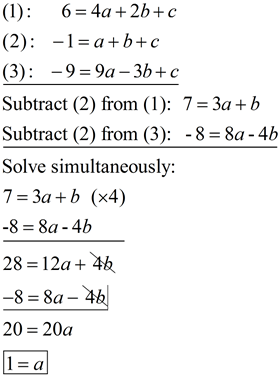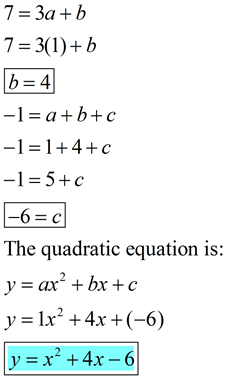You can read more about solving three equations with three unknowns (as seen above)
under the Polynomial section.Can you use the sum and product of the roots formulas to find an equation?
From the Nature of Roots page, we know that given the roots of a quadratic equation,
we can find an equation by using the information about the sum and product of the roots.
 6. Determine an equation that has the solutions x = -4 and x = 3. ax2 + bx + c = 0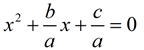Sum of roots = -b/a = (-4 + 3) = -1;    b/a = +1 Product of roots = c/a = (-4)•(3) = -12 ANSWER: x2 + 1x + (-12) = 0     or    x2 + x - 12 = 0 Remember, as we have seen in the examples above, this is ONE possible equation.Can you use the graphing calculator to create a quadratic equation?
 7. When three or more points are known, the quadratic regression option on the graphing calculator can be used to create a quadratic "model" for the data. Refer to Quadratic Regression Model Example for directions on using the calculator for a quadratic regression.In summary: If you know the vertex and a point on a parabola, use the "vertex-form", y = a(x - h)2 + k, to write the equation of the parabola. If you know three points on the parabola, but not the vertex, use the form y = ax2 + bx + c   to write the equation of the parabola.